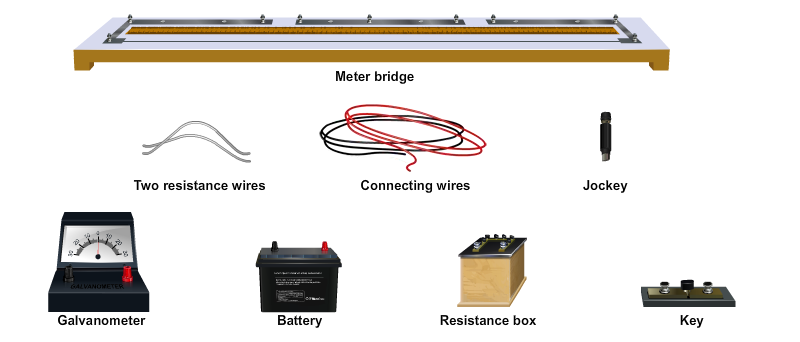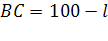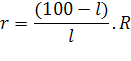Equivalent Resistance of Resistors (Series)

# Materials Required:# Procedure:

## As performed in the real lab:

1. Make a circuit as shown in the figure.
2. Ensure that all the connections are tight.
3. Check whether the connections are correct by removing some resistance from the resistance box and close the plug key ‘K’.
4. Press the jockey at the left extreme of the metre bridge. Mark the direction of the deflection on the galvanometer. Again press the jockey at right extreme of the metre bridge and mark the direction of the deflection again. If the directions of the deflections are in opposite direction, then the connections are correct, otherwise check the connections.
5. The balancing point is obtained by sliding the jockey along the wire and when there is no deflection in the galvanometer.
6. Take at least three sets of observations by moving the jockey from both the end of the bridge for each wire and also when it is serially connected.
8. Find its resistance of this combination as mentioned in the below observation table.

## As performed in the simulator:1. Your simulator will consist of a metre bridge kept on a table, battery, resistance box and wires on the side bar menu.
2. You can calculate the resistance of a single wire or serially connected wire by selecting from the drop down menu, “Arrangement of Resistors”.
3. If you selected, “Single”, then drag the battery and the resistance box shown on the side bar menu near to the metre bridge using your mouse.
4. Drag one of the wires to the right gap of the metre bridge.
5. Now the button, “Start experiment” will be enabled.
6. Now you can select your desired resistance from the resistance box just by clicking on the box and then choosing the resistance from the pop-window, “Select Resistance”. Now close the pop-window.
7. Click on the enabled button and "Insert Key”.
8. Now you can move the jockey from one left end to right either by moving the jockey with your mouse or by moving the slider, “Jockey Position”.
9. Simultaneously check the readings of the galvanometer, once the needle reaches the zero reading, stop moving the jockey and note down the length of the wire from the balanced position on the left side, let say “AB” which is l cm.
10. Repeat the same by moving the jockey from the right end to the left and note down the length of the wire from the balanced position on the right side, let take it as “BC” which is 100-l cm.
11. Repeat the same procedure with second wire and note down the lengths.
12. For each wire take three readings and calculate its mean readings/resistance.
13. Repeat the same procedures for the series connection.

# Observations:

Table for Length (l) and Unknown Resistance (X)

 Resistance coil Serial No. of Obs. Resistance from the resistance box R (ohm) Length(cm) Length(cm) Resistance(ohm) Mean resistance (ohm) r1 only 1. 2. 3. r1 =........... r2 only 1. 2. 3. r2 =........... r1 and r2 in series 1. 2. 3. Rs =...........

# Calculations:

1. Calculation for r1 only, r2 only, r1 and r2 in series

2. Calculation for verification of laws in series

Experimental value of Rs               = ........... ohm
Theoretical value of Rs       =   r1 + r2 = ........... ohm
Difference (if any)                        = ........... ohm

# Result:

Within the limits of experimental error, experimental and theoretical values of Rs are same. Hence, law of resistance in series is verified.